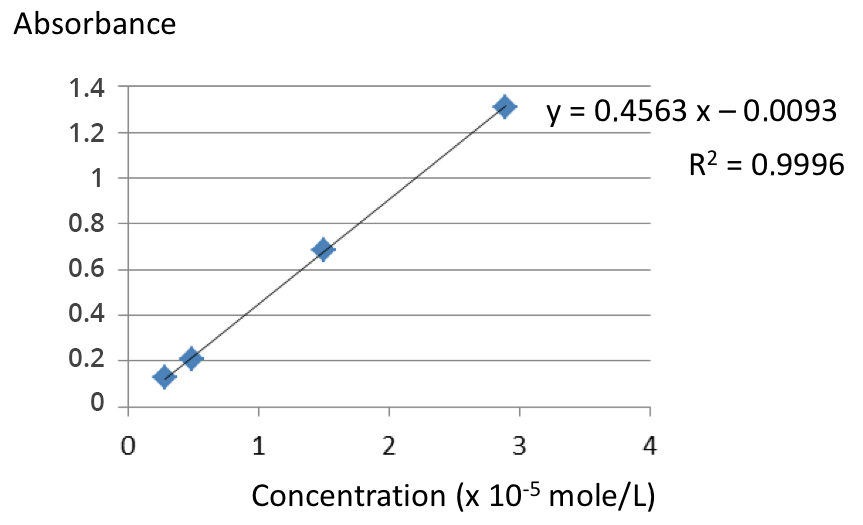## Exercise 2: Sample measurement based on the calibration

The calibration range of Acid Black 1 dye was analyzed by spectrophotometry at 618 nm (maximum absorbance of this dye). The calibration curve obtained is given below:Question 1: For a water sample containing this dye, an absorbance of 1.67 is measured – can we use this calibration line for this sample?Question 2: Why?

Answer: The value is outside the calibration range.Question 3: How can the dye concentration of this sample be estimated?

Answer: By diluting the sample in order to bring it within the calibration range.Question 4: For another water sample containing this dye, an absorbance of 0.264 is measured - what is the dye concentration (expressed in µmol/L) of this sample?